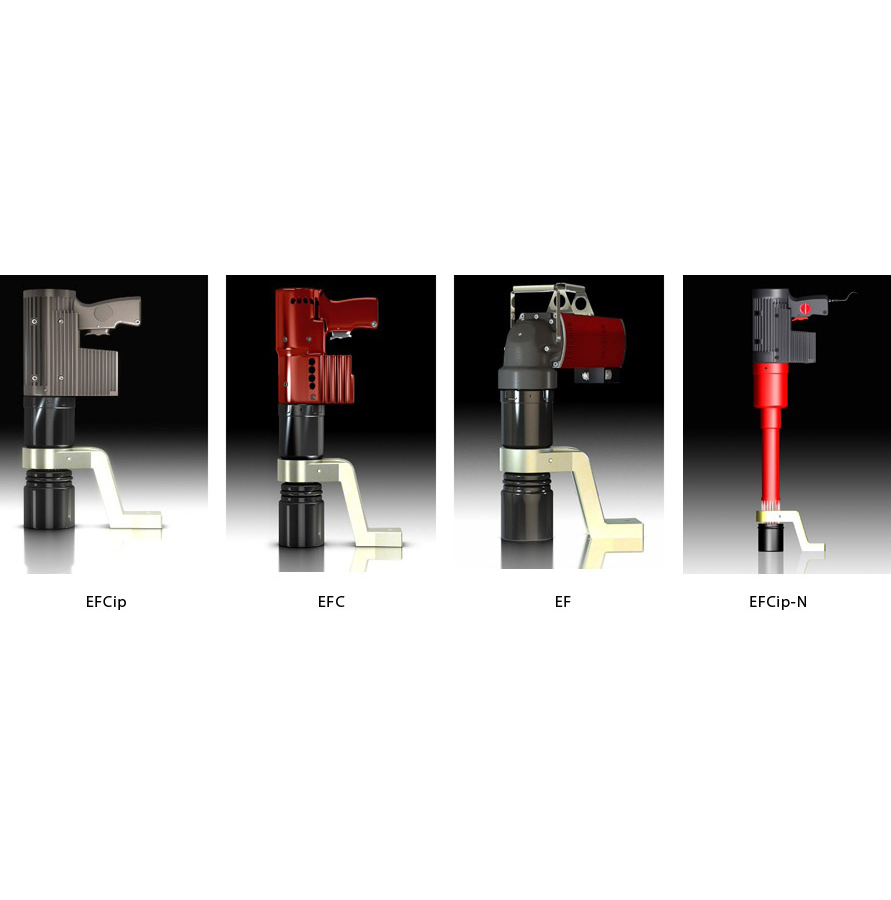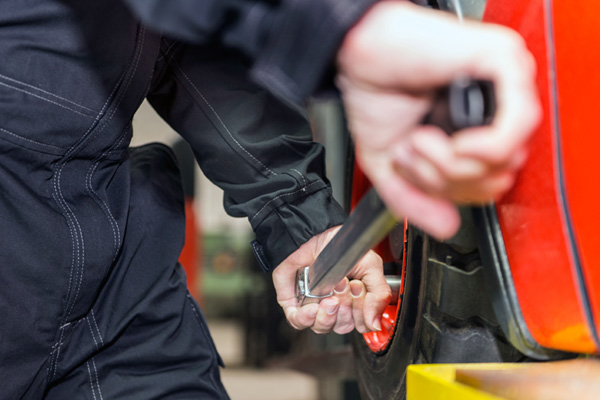Bolting

Correct Technique Using Torque Multiplier (Torque Multiplier)

Created By: Hyprowira | Published Date: 02 July 2017 | Last Modified: 17 December 2019

The machine-making industry is becoming a highly used industry today because it brings so many things that are needed for the wider community. By making machines in the industry, the machines that are made can be useful for use. To be able to strengthen the production of machinery, other supporting tools are needed. One of them is the multiplier torque. Torque multiplier is a very useful tool for the machine manufacturing industry because its main function is that it can increase torque levels in an easier way in areas that cannot be reached by human hands. The use of multiplier torque is usually used to overcome the bending caused by mounting bolts or nuts with a different firmness.

Of the many brands of multiplier torque on the market today, Alkitronic is one of the brands that sell a variety of torque multiplier products that have been sold worldwide and are superior among other brands. The advantages of the Alkitronic brand in producing multiplier torque are the quality of power and also the use of a better, more efficient, providing a better level of firmness so that the resulting torque output can be more precise, consisting of many variations of power sources and of course using technology from Germany that has patented and tested for wind turbine applications.

That's a brief explanation of the superiority of the multiplier torque from the proven Alkitronic brand quality. With the need for multiplier torque in the engine manufacturing industry, and also the importance of the existence of a multiplier torque in order to facilitate the machining system as well, there is a variety of interesting information about the multiplier torque that you can know about. For those who are curious about other interesting information about multiplier torque, the following is a further explanation of the function and benefits of multiplier torque in the engine industry and how to use the correct techniques to be more efficient, effective and optimal.

Torque Multiplier Function

From the explanation above, actually there is little information about the function of the multiplier torque itself. In general, the multiplier torque has a function to double the ratio of the torque value on an engine, so the greater the value of the torque ratio, the easier the engine is run which is adjusted to the load that is borne.

So when the torque value has been multiplied using a multiplier torque, the power generated is also greater. This is the reason why multiplier torque is needed by the machine manufacturing industry. The heavy burden borne by industrial machines in the manufacturing process can be made lighter with the use of multiplier torque.

In use, the multiplier torque is often referred to as cheater bars and is considered safer to use to increase the torque value compared to using extended handles technically. The teeth that are often used for techniques using multiplier torque are epicyclic gearing where at each stage the gearing will increase the torque level with the help of multiplier torque.

Along with the use of a multiplier torque, the output ratio of the engine speed produced is smaller when compared to the input. The decrease in speed in the mechanism of this engine actually makes it more proportionate to the value of the increase in torque. For example, using a torque multiplier in a ratio of 3: 1, in theory, makes the torque level tripled to 3 times but the speed is only 1/3 of it. Even so, in actual practice, the output of the torque value that comes out is not as much as the theoretical calculation due to friction in the engine and also other inefficiencies in the engine mechanism. That way the ratio of torque output to engine speed is still proportional.

That is an explanation of the function of the multiplier torque that makes the industrial machinery making machines work more optimally. Indeed, in the beginning, the multiplier torque will not be too used to increase the torque value of the engine. But over time and also the addition of an increasingly heavy production load, the multiplier torque becomes very useful too. This multiplier torque function is highly used for the industrial bolting machine. Now it's time to go into the procedures for using a multiplier torque.

How to Use Torque Multiplier?

After explaining the function of the torque multiplier in the previous point, now is the time to enter into the discussion on how to use the multiplier torque. To be able to use the multiplier torque, precise and regular steps are needed. Do not until when you use the multiplier torque, you actually take the wrong step. Instead of being able to increase the torque value of the industrial machine-making machine, the existing one even damaged the engine and the torque value did not go up. To be able to use the multiplier torque, then consider how to use it below:

First Step

The first step to using a torque multiplier is to make sure you read and understand the manual that is included in every package of purchase of the torque multiplier including the warnings given. Look at the list of specifications for the value of the multiplier comparison. For example, the multiplier torque that you have has a ratio of 25: 1, meaning you have to set the moment key used at a value of 1/25 of the required torque. Do not set more than the recommended input value.

Second Step

The second step is to choose the appropriate reaction point. A good reaction point position will produce maximum pressure. Meanwhile, if the wrong choice of reaction point then the resulting pressure can make the mechanism become damaged or worn. After the reaction point is right, start to tighten the moment lock and also the multiplier torque until you hear a click, which means the torque multiplier is safely locked and can be used.

That is an explanation of how to use the torque multiplier appropriately and effectively. But before following how to use the multiplier torque as explained above, there are a few things you should know first. There are several usage warnings that must be considered when using a multiplier torque, it is better not to use levers that are too long. Although it looks easier to use, using a long lever can be dangerous if it is coupled with a multiplier torque.

To release the multiplier torque is done by reversing the method of installation, do not immediately remove or even forced. Besides using the multiplier torque product from Alkitronic, choosing the right moment lock will also make your mechanical work easier and more cost-efficient.

So from the explanation of the things that must be considered when using a multiplier torque, it is clear that the multiplier torque product from Alkitronic can be the best choice for you. You don't want to use another brand's multiplier torque, which is still below the quality of the Alkitronic multiplier torque. Instead of getting a product that is of worse quality and strength, don't hesitate to choose the multiplier torque from Alkitronic.

Use Torque Multiplier Safely

The above is already explained about how to use the multiplier torque. But in order to get a clear way to use a multiplier torque, then the methods below you need to know and need to be followed as well. Here's a brief explanation:

- When the multiplier is used in a regular frequency it should not need to be used then stored again, but left alone in standby. But if it needs to be stored, leave a little compression on the multiplier torque, don't get to zero and don't be too full because it can interfere with the accuracy of the torque needed.

- In using a torque multiplier it should be done carefully, carefully, and just one click away. Each additional click is used then there will be an additional torque on the tool that is done.

- The multiplier torque used for steering the left hand on certain vehicles and on the left pedal of the bicycle uses a spin that is counterclockwise, so before using it should check the specifications first because if you turn wrong then you can lose control of the torque.

- There are many smartphone applications that can be used to convert units used in torque to be more precise.

- When there are tools that will be worked with multiplier torque, adjust the scale to the required torque specifications.

Mechanism Using Multiplier Torque

Next is an explanation of the mechanism using multiplier torque. It's actually already explained above, but when you read the manual given by the torque multiplier manufacturer, it might be a little inconvenient for you. Take it easy, we have summarized the exact mechanism of using a multiplier torque that is simpler and easier for you to follow and understand. Here are a few simple steps to use this tool:

- Position the hand in the middle of the marked area or the load point area, this is to increase the level of precision in adding torque to the tool.

- The torque multiplier can be used to remove bolts or nuts as long as the torque scale used does not exceed the maximum limit.

- It is strictly forbidden to add an extension of the handle, for example, using pipes, because it can reduce accuracy.

- Not all multiplier torque has a safety lock, but if there is do not ignore its use.

- There are several types of parts outside the multiplier equipment from the manufacturer that can be used to operate the multiplier more easily. For example fittings with bird leg shapes that can be used to install bolts that are box-shaped.

Those are some things you should know about the multiplier torque which includes how to use it safely and also the things that must be considered when working with a multiplier torque. With the complete information about the multiplier torque above, you certainly know about how important the multiplier torque is for the machine-making industry which results from industrial production is useful for the community.

With the many uses of the machine produced, it is needed for an industrial machine production tool that has qualified quality. It was there that the multiplier torque was able to complete the business of increasing the torque value of the industrial machine.

9125 views

Other Articles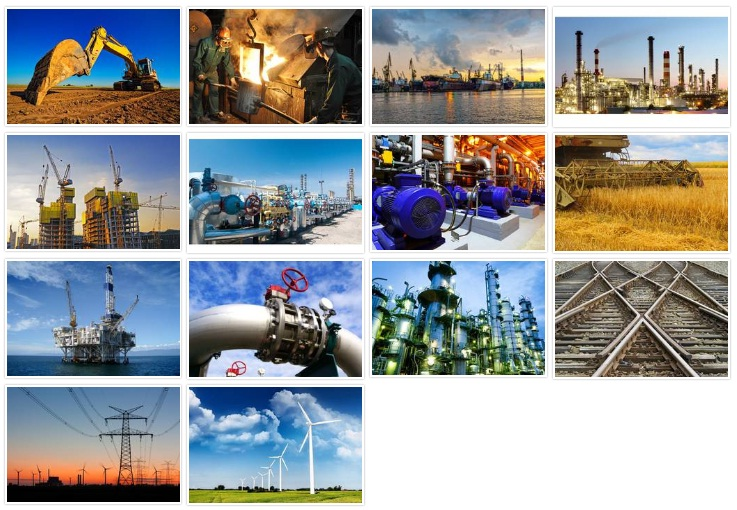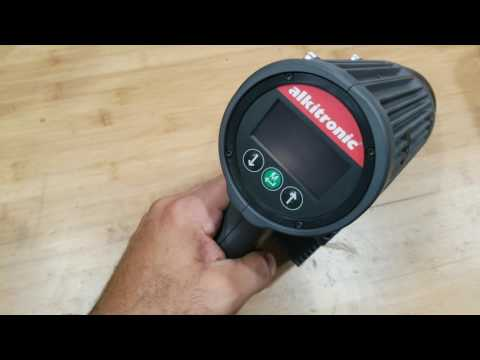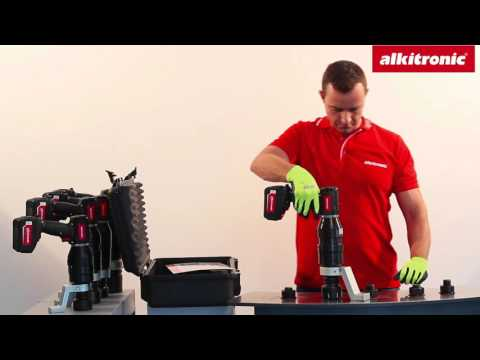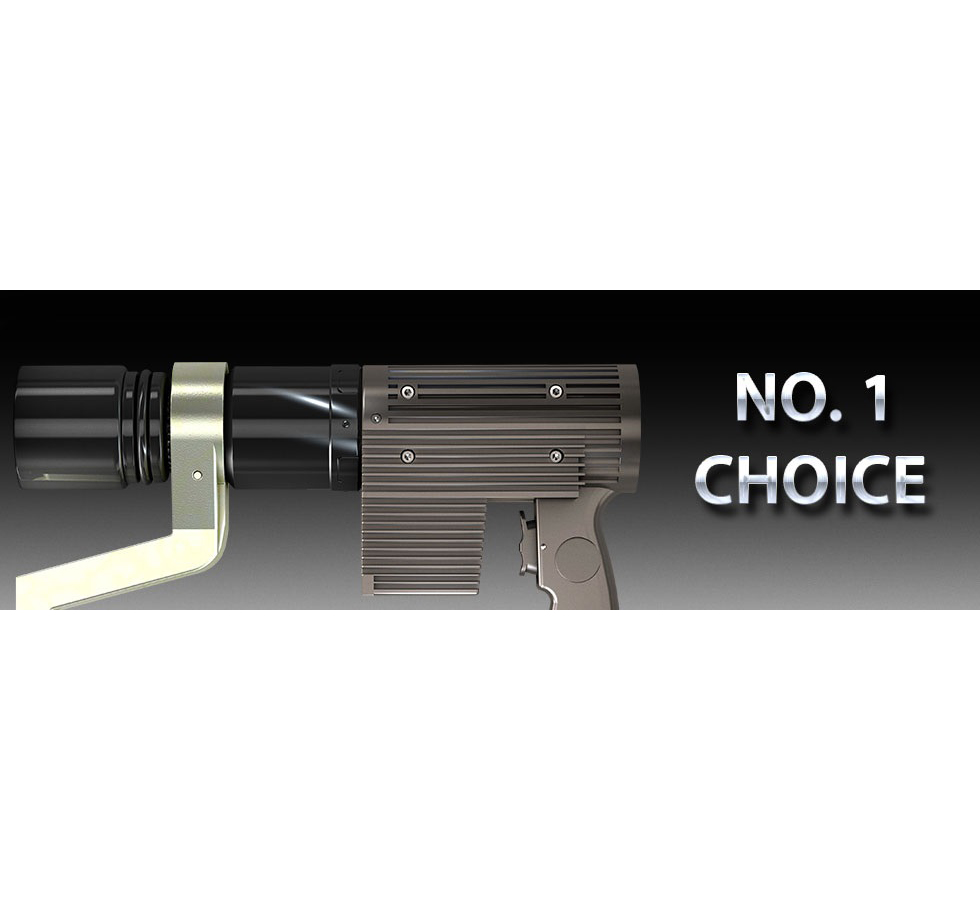12 September 2017